# Solution assignment 17 Quadratic equations (factorizing)

### Assignment 17

Try to solve by factorizing: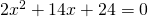### Solution

In this equation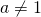, but both sides can be divided by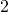without fractions as coefficients as a result:We have to find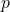and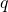such that: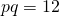and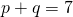The following values will do: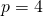and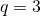Thus the equation can be written as: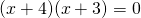and thus the solutions are: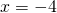or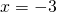0